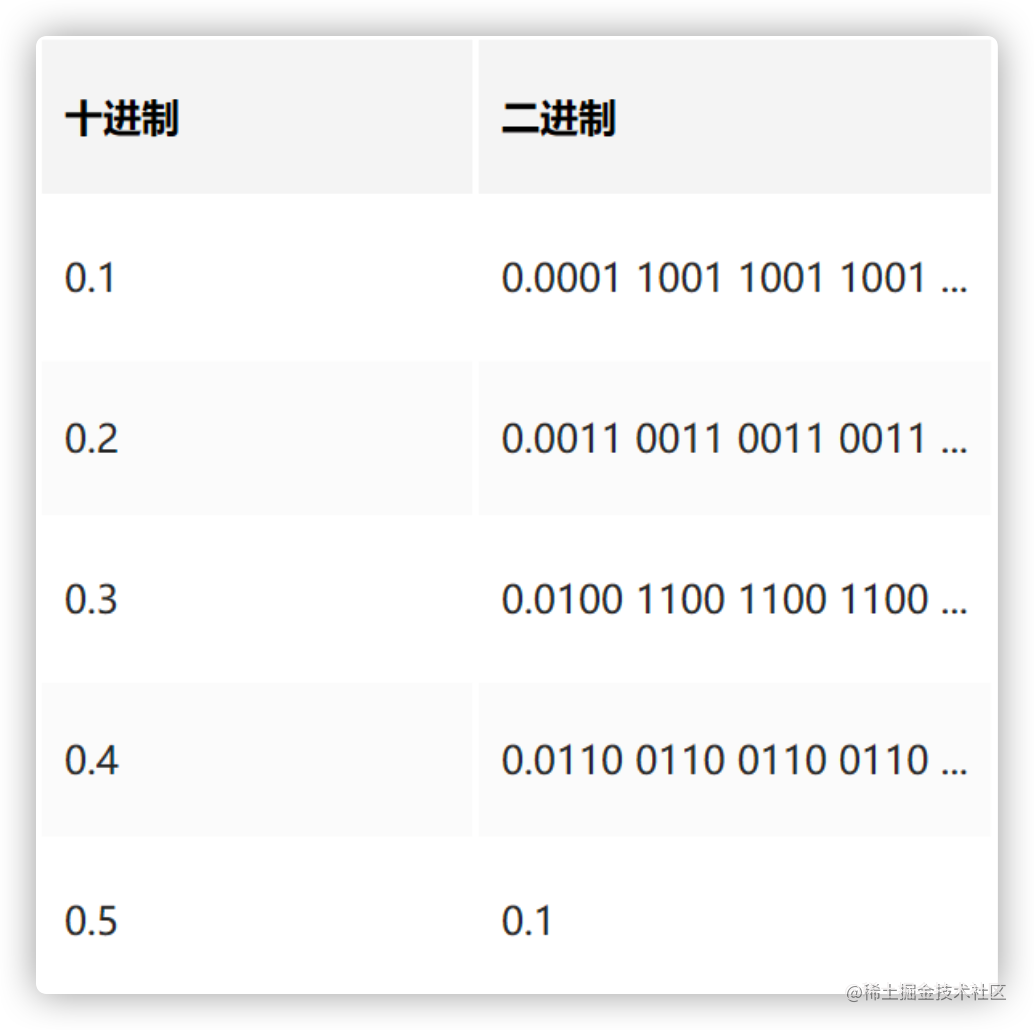JS计算精度问题解决

出现的情况

// 加法
0.1 + 0.2 = 0.30000000000000004
// 减法
1.5 - 1.2 = 0.30000000000000004
// 乘法
0.8 * 3 = 2.4000000000000004
// 除法
0.3 / 0.1 = 2.9999999999999996

问题的原因• 产生浮点数计算精度不准确的原因: 在计算机角度，计算机算的是二进制，而不是十进制。二进制后变成了无线不循环的数，而计算机可支持浮点数的小数部分可支持到52位，所有两者相加，在转换成十进制，得到的数就不准确了，加减乘除运算原理一样。
• 通俗的解释: 比如一个数 1÷3=0.33333333...... 大家都知道3会一直无限循环，数学可以表示，但是计算机要存储，方便下次取出来再使用，但0.333333...... 这个数无限循环，你让计算机怎么存储?计算机再大的内存它也存不下，对吧! 所以不能存储一个相对于数学来说的值，只能存储一个近似值，所以当计算机存储后再取出来用时就会出现精度问题。

解决方案 - Math.js

// 封装
import math from 'mathjs';
export default {
// 加
},
// 减
subtract(num1,num2){
return math.subtract(math.bignumber(num1),math.bignumber(num2));
},
// 乘
multiply(num1,num2){
return math.multiply(math.bignumber(num1),math.bignumber(num2));
},
// 除
divide(num1,num2){
return math.divide(math.bignumber(num1),math.bignumber(num2));
},
}

// 使用
import math from './math.js';